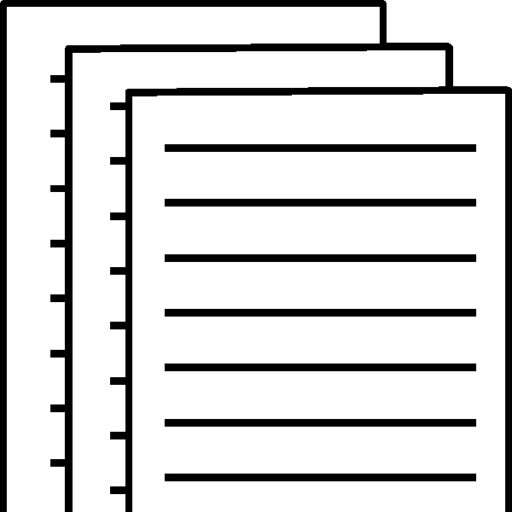## What have you learned about statistics

What have you learned about statistics?

BUS 308 Statistics for Managers

Introduction

Before beginning this course I had very little knowledge about statistics and what it entailed. Statistics is defined in Merriam-Webster dictionary as, “a branch of mathematics dealing with the collection, analysis, interpretation, and presentation of masses of numerical data”. Which I can verify, As I have learned through this course many mathematical formulae are used for statistics including; mean, median, and mode. In this paper I will outline five topics I have learned over the last five weeks.

Descriptive statistics

According to Will Kenton, “Descriptive statistics are brief descriptive coefficients that summarize a given data set, which can be either a representation of the entire or a sample of a population.” Which basically means, descriptive statistics of what is happening in the data set that is being tested. From these large data sets, patterns can be discerned. The reason descriptive statistics are important is because it allows us to see the data in a more meaningful way. The negative aspect of descriptive statistics is that it cannot be applied as a generalization, but only applied to the data set it contains.

Inferential statistics

Inferential statistics enable the user to make generalized statements about the samples that have been collected. It is crucial that the sample collected for inferential statistics accurately represent the population. Several inferential statistics are used in correlation, regression, and confidence intervals. Chi-square, t-test, and Analysis of variance (ANOVA) can be used to determine a samples relevance. The negative aspect of inferential statistics is that the results are based on a population which is not fully measured.

Hypothesis development and testing

Testing a hypothesis is different than just asking a question. Hypothesis are normally very targeted and specific research questions that would also generally make a prediction. Developing a hypothesis begins with forming a question around sets of variables. John Mcdonald, author of the Handbook of Biological Statistics describes testing a hypothesis as, “ It involves testing a null hypothesis by comparing the data you observe in your experiment with the predictions of a null hypothesis. You estimate what the probability would be of obtaining the observed results, or something more extreme, if the null hypothesis were true. If this estimated probability (the P value) is small enough (below the significance value), then you conclude that it is unlikely that the null hypothesis is true; you reject the null hypothesis and accept an alternative hypothesis.” Although there are many different techniques of doing hypothesis testing, In our class we used statistical testing which is slightly different than biological testing.

Selection of appropriate statistical tests

As we learned in the first week of the course, selecting the proper analysis for the data is crucial. Variables can come in the form of intervals, nominal, rank-ordered, or ratio-level. The data scientist would want to start by understanding what the question is asking in order to use the correct statistical test. For instance in week two we learned the F-tests used to determine variances in interval data, and T-Tests, used to determine differences in the means of a sample or group after the variances are known.

Evaluating statistical results

Once the data is arranged and sorted properly, the variables can be applied to their test. The user would then have a new sheet appear with the result of the test. These results can be used to see if the hypothesis is null. If the results indicate reinforcement to the hypothesis, then the test was successful, if not then the test may need to be rerun with a larger sample size or more truthful answers.

Resources

To view and download a complete answer, scroll down to the bottom to pay